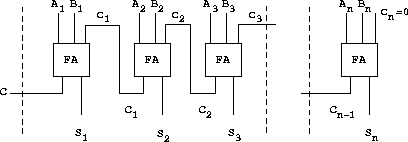Figure 3.27 shows a ripple-carry adder formed by stringing together n full-adders. This is the simplest form of parallel adder for adding two n-bit binary numbers. The inputs A₁, A₂, A₃, ..., Aₙ and B₁, B₂, B₃, ..., Bₙ are the two binary numbers to be added (each Aₖ and Bₖ is a 0 or a 1). The circuit generates S₁, S₂, S₃, ..., Sₙ, the n bits of the sum, and C, the carry from the addition. Write a procedure `ripple-carry-adder` that generates this circuit. The procedure should take as arguments three lists of n wires each -- the Aₖ, the Bₖ, and the Sₖ -- and also another wire C. The major drawback of the ripple-carry adder is the need to wait for the carry signals to propagate. What is the delay needed to obtain the complete output from an n-bit ripple-carry adder, expressed in terms of the delays for and-gates, or-gates, and inverters?